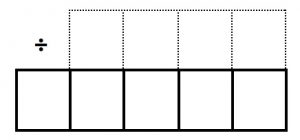A game for any number of players.  You want to win so THINK MATHEMATICALLY!

For the TARGET 1000 version you divide a 4-digit number by a 1-digit number. Think about aiming for an answer near 1000, so how do you choose the thousands digit and the single number?

For the simpler TARGET 100 version you divide a 3-digit number by a 1-digit number. Think about aiming for an answer near 100, so how do you choose the hundreds digit and the single number?

You will need a set of 10 cards numbered 0 to 9 or a spinner.

VERSION 1 This is a game of chance.

Each player must draw a grid on a showboard or in their notebook. Draw the grid as shown for dividing a 4 digit number by a 1 digit number and use 4 squares rather than 5 for dividing a 3 digit number by a 1 digit number.

Spin the spinner and the players must write the digit in one of the five boxes. Once a number is written the position cannot be changed.

Repeat until you have a number in every box.

Then the learners divide the bigger number by the 1-digit number and write the answer on the top line.

The winner is the player (or players) with the quotient closest to 100 for the 3-digit number divided by a 1-digit number or closest to 1000 for the 4-digit number divided by a 1-digit number.

Score 1 point for a win.

VERSION 2 This is a game of skill

Each player must draw the grid on a showboard or in their notebook.

Spin the spinner 4 (or 5) times and make a note of the numbers that come up. The players must write the digits in the boxes so that the answer to the division is as close as possible to 100 for the 3-digit number divided by a 1-digit number or closest to 1000 for the 4-digit number divided by a 1-digit number.  A time limit such as 5 minutes is set for this. The player (or players) with the quotient closest to100 (or 1000) wins. Score 1 point for a win.

Alternatively either version of the game can be played by pairs of learners ain the same way and they will need a spinner for each pair.

Tagged with: